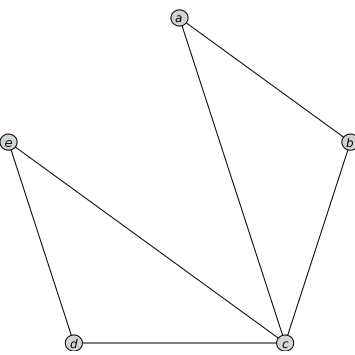Quiz
 generate an interactive quiz

 Calling Sequence Quiz( question_statement, grader, init, options ) Quiz( MapleTAImport )

Parameters

 question_statement - string or module grader - procedure or value init - procedure or value MapleTAImport - Record imported by MapleTA:-Import

Options

 • style = math, truefalse, multiplechoice, or multipleselect
 The style option specifies the kind of question to display.
 • scramble = true or false
 The scramble option applies only to multiple choice and multiple select style questions.  The order of the entries are displayed in a random order.  The default is true.
 • mathsize = [width,height]
 This size will be used to format the answer input box.
 • plotsize = [width,height]
 This size will be used to format the size of any plot that appears in the question.
 • numchoices = integer
 This can be used to specify the maximum number of choices in a multiple-choice question if there could be more than five choices and it is not obviously determined by the initialization data.
 • inertform = true or false
 When set to true, values returned from an answer area and sent to the grading routine will use the InertForm format to prevent automatic simplification.  This option only applies to the implicitly presented output area and response math container.  It does not affect Get or Set values.
 • name = string
 This option is used to give the question a name when used in conjunction with output = mapleta
 • output = quiz, module, or mapleta
 The output option determines the return behavior of the Quiz command.  The default is to generate an interactive quiz.  Choosing output = module will return a Record structure encapsulating the data used to drive the interactive quiz.  The third option, output=mapleta will cause a Record structure to be returned in such format that is suitable for giving to the MapleTA:-Export command so the quiz can be used in Maple T.A.
 • The following options pertain to generating multiple questions by passing in an array of modules generated by calling the Quiz command with the 'output'=module option:
 • numberformat = identical(roman,lowercaseroman,none,decimal) := decimal,
 When displaying an array of questions each question is numbered using decimal numbers by default.  Optionally roman numerals or no numbering can be chosen.
 When displaying an array of questions, the text style used to display the introductory numbered heading can be one of these styles.
 • numberprefix = string := Question ,
 The prefix in front of a numbered question can be specified with the numberprefix option.
 • border = truefalse := false
 A border can be optionally turned on around each question when displaying an array of questions.

Description

 • The Quiz command generates a simple interactive tool that displays a question, accepts a response, and grades the response. If the question generation is algorithmic, then it also offers the ability to try another version of the question with new random variables.
 • The question_statement should be a string containing the text of the question.  Blanks can be implicitly assigned by including "$" variables within the question text. The first argument can also be a previously generated question module or array of question modules built using the Quiz command with the option 'output'=module  • The grader argument is either the correct answer, or a procedure that returns true or 1 for a correct answer and false or 0 for an incorrect response.  The grader procedure should either take two arguments -- the response and expected answer -- or use Quiz:-Get to get the "$RESPONSE" variable.
 • The init argument is either an initial value or a procedure that generates initial values.  For multiple choice questions init can be a list of choices.
 As a procedure, init takes no arguments. It can either set "\$" variables using Quiz:-Set, or return a value that will be implicitly filled into the question.

Examples

The Quiz Example Worksheet provides a number of examples with detailed explanations.   A few simple questions are reproduced here.

In order to test the following examples, make sure you open the help page as a worksheet.  The components are not interactive when viewed within the help system.

 > $\mathrm{with}\left(\mathrm{Grading}\right):$
 > $\mathrm{Quiz}\left("Is 1+1=2?",\mathrm{true},'\mathrm{style}'=\mathrm{truefalse}\right)$

 Is 1+1=2?> Quiz("Is the following number prime?", true, proc(r) nextprime(rand(r..r+20)()) end proc, 'style' = truefalse, 1000);

 Is the following number prime?> init := proc ()     local fn, n, choices;     choices := [sin(x), cos(x), tan(x)];     n := rand(1..numelems(choices))();     fn := choices[n];     Quiz:-Set(= fn);     [plot(fn), plot(-fn), plot(Pi*fn, x = -2*Pi .. 2*Pi)]; end proc:
 > $\mathrm{Quiz}\left("Which is a plot of \left\{FUNC\right\}?",1,\mathrm{init},\mathrm{style}=\mathrm{multiplechoice},\mathrm{plotsize}=\left[250,150\right]\right)$

Which is a plot of ?The following example shows how to generate and display multiple questions from the same procedure, using the output=module option.  Note that since module is a keyword, it needs back-ticks around it in order to parse as a name.

 > $A≔\mathrm{Array}\left(\right):$
 > $A\left(1\right)≔\mathrm{Grading}:-\mathrm{Quiz}\left("Is 1 + 1 = 2?",\mathrm{true},'\mathrm{style}'=\mathrm{truefalse},'\mathrm{output}'=\mathrm{module}\right):$
 > $A\left(2\right)≔\mathrm{Grading}:-\mathrm{Quiz}\left("What is 1 + 1?",2,'\mathrm{style}'=\mathrm{math},'\mathrm{output}'=\mathrm{module}\right):$
 > $A\left(3\right)≔\mathrm{Grading}:-\mathrm{Quiz}\left("Pick C.",3,\left["A","B","C"\right],'\mathrm{style}'=\mathrm{multiplechoice},'\mathrm{output}'=\mathrm{module}\right):$
 > $A\left(4\right)≔\mathrm{Grading}:-\mathrm{Quiz}\left("Which of the following could be portions of the plot of sin\left(x\right)?",\left\{1,2\right\},\left[\mathrm{plot}\left(\mathrm{sin}\left(x\right),x=0..\mathrm{rand}\left(2..3\right)\left(\right)\right),\mathrm{plot}\left(\mathrm{sin}\left(x\right),x=\mathrm{rand}\left(2..3\right)\left(\right)..5\right),\mathrm{plot}\left(2\mathrm{sin}\left(x\right),x=\mathrm{rand}\left(2..3\right)\left(\right)..\mathrm{rand}\left(4..7\right)\left(\right)\right)\right],\mathrm{style}=\mathrm{multipleselect},\mathrm{gradebyindex}=\mathrm{true},\mathrm{size}=\left[250,150\right],'\mathrm{output}'=\mathrm{module}\right):$
 > $\mathrm{Grading}:-\mathrm{Quiz}\left(A,\mathrm{numberformat}=\mathrm{lowercaseroman},\mathrm{numberprefix}="Question 1.",\mathrm{border}=\mathrm{true}\right)$

Question 1.i

 Is 1 + 1 = 2?Question 1.ii

 What is 1 + 1?Question 1.iii

Pick C.Question 1.iv

Which of the following could be portions of the plot of sin(x)?> 

Compatibility

 • The Grading[Quiz] command was introduced in Maple 18.